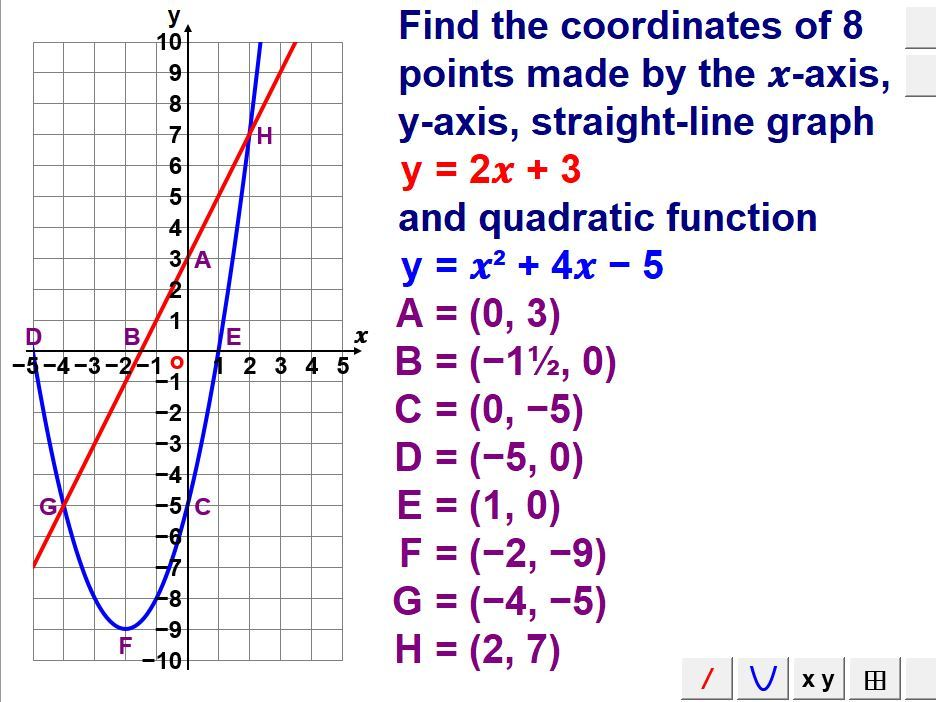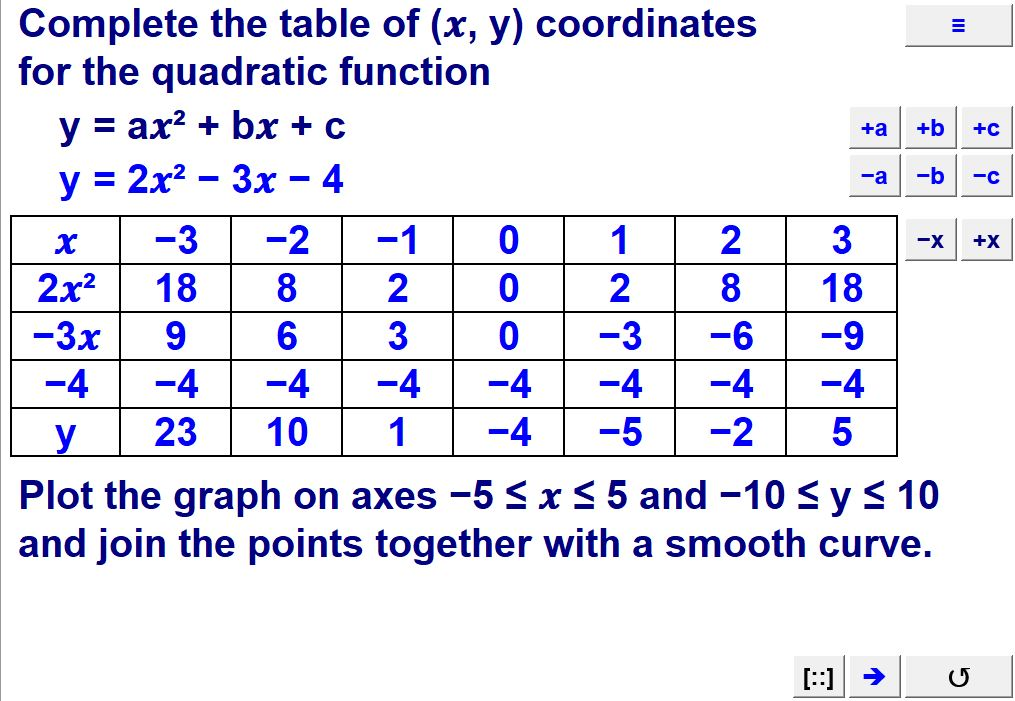Last updated

2 February 2021

#### Share thisxlsm, 3.08 MB

Interactive macro-enabled MS-Excel spreadsheet. Contents: Plot graphs of quadratic functions y = ax² + bx + c (7× by partⁿ). Plot graphs of quadratic functions y = ax² + bx + c (7× by substⁿ). Plot graphs of quadratic functions y = ax² + bx + c (11× by partⁿ). Plot graphs of quadratic functions y = ax² + bx + c (11× by substⁿ). Plot graphs of cubic functions y = ax³ + bx² + cx + d (11× by partⁿ). Plot graphs of cubic functions y = ax³ + bx² + cx + d (11× by substⁿ). Draw two or three graphs of quadratic or cubic functions (integers). Draw two or three graphs of quadratic or cubic functions (fractions). Find two exact roots of a quadratic function y = (ax + b)(cx + d). Find three exact roots of a cubic function y = (ax + b)(cx + d)(ex + f). Find coordinates of 8 points formed by a linear & quadratic graph. Match different graphs on the same axes to one of five functions. Apply 4 different transformations to a graph of a quadratic function.

### Reviews

This resource hasn't been reviewed yet

To ensure quality for our reviews, only customers who have purchased this resource can review it

Report this resourceto let us know if it violates our terms and conditions.
Our customer service team will review your report and will be in touch.

£1.00